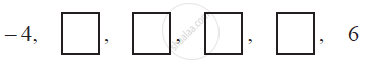Share

# In the following APs, find the missing terms in the boxes - CBSE Class 10 - Mathematics

#### Question

In the following APs, find the missing terms in the boxes :#### Solution

For this A.P.,

a = −4 and

a6 = 6

We know that,

an = a + (n − 1) d

a6 = a + (6 − 1) d

6 = − 4 + 5d

10 = 5d

d = 2

a2 = a + d = − 4 + 2 = −2

a3 = a + 2d = − 4 + 2 (2) = 0

a4 = a + 3d = − 4 + 3 (2) = 2

a5 = a + 4d = − 4 + 4 (2) = 4

Therefore, the missing terms are −2, 0, 2, and 4 respectively.

Is there an error in this question or solution?

#### APPEARS IN

NCERT Solution for Mathematics Textbook for Class 10 (2019 to Current)
Chapter 5: Arithmetic Progressions
Ex. 5.20 | Q: 3.4 | Page no. 106
Solution In the following APs, find the missing terms in the boxes Concept: nth Term of an AP.
S